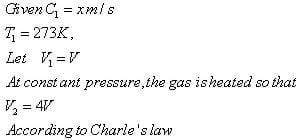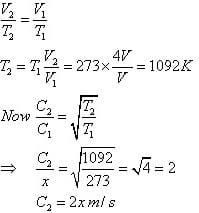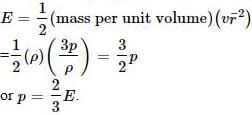NEET  >  Test: Kinetic Theory - 1

# Test: Kinetic Theory - 1

Test Description

## 10 Questions MCQ Test Physics Class 11 | Test: Kinetic Theory - 1

Test: Kinetic Theory - 1 for NEET 2022 is part of Physics Class 11 preparation. The Test: Kinetic Theory - 1 questions and answers have been prepared according to the NEET exam syllabus.The Test: Kinetic Theory - 1 MCQs are made for NEET 2022 Exam. Find important definitions, questions, notes, meanings, examples, exercises, MCQs and online tests for Test: Kinetic Theory - 1 below.
Solutions of Test: Kinetic Theory - 1 questions in English are available as part of our Physics Class 11 for NEET & Test: Kinetic Theory - 1 solutions in Hindi for Physics Class 11 course. Download more important topics, notes, lectures and mock test series for NEET Exam by signing up for free. Attempt Test: Kinetic Theory - 1 | 10 questions in 10 minutes | Mock test for NEET preparation | Free important questions MCQ to study Physics Class 11 for NEET Exam | Download free PDF with solutions
 1 Crore+ students have signed up on EduRev. Have you?
Test: Kinetic Theory - 1 - Question 1

### At absolute zero temperature may be defined as that temperature at which

Detailed Solution for Test: Kinetic Theory - 1 - Question 1

At 0K temperature we know that there is no molecular motion, that is the KE of the particles gets 0. Thus we can say the combined KE of a gaseous system is zero, but as there combined mass cant be zero thus the combined of the square of velocities of the particles is zero, which means that the root mean square velocity of the gas is zero.

Test: Kinetic Theory - 1 - Question 2

### Pressure exerted by an ideal gas molecule is given by the expression

Test: Kinetic Theory - 1 - Question 3

### If the r.m.s speed of oxygen at NTP is x m/s. If the gas is heated at constant pressure till its volume is four fold, what will be its final temperature and r.m.s speed?

Detailed Solution for Test: Kinetic Theory - 1 - Question 3Test: Kinetic Theory - 1 - Question 4

The perfect gas equation is PV = nRT where n is the

Detailed Solution for Test: Kinetic Theory - 1 - Question 4

n is the number of moles.

Test: Kinetic Theory - 1 - Question 5

In a mixture of ideal gases at a fixed temperature the heavier molecule has the lower average speed. This is easiest to conclude from the equation

Detailed Solution for Test: Kinetic Theory - 1 - Question 5

In option A, v is inversely proportional to mass. Hence, the condition of the question is seen accurately in that option.

Test: Kinetic Theory - 1 - Question 6

According to kinetic theory of gases, 0K is that temperature at which for an ideal gas

Detailed Solution for Test: Kinetic Theory - 1 - Question 6

According to kinetic theory of gases, 0K is that temperature at which for an ideal gas the internal energy is zero because at 0K nearly all molecular motion stops.

Test: Kinetic Theory - 1 - Question 7

The kinetic energy of one mole of an ideal gas is E=3/2 RT. Then Cρ will be

Detailed Solution for Test: Kinetic Theory - 1 - Question 7

We know that,
Cp=cv+R
E=3Rt/2
also,
E=Cv
cp=R+3RT/2
=5/2 RT
=2.5R

Test: Kinetic Theory - 1 - Question 8

Value of gas constant, R for one mole of a gas is independent of the

Detailed Solution for Test: Kinetic Theory - 1 - Question 8

We know that PV=nRT also PM=dRT
So in the equation The value of R depends on P , V , n , T , d , M
except atomicity
so the ans is A

Test: Kinetic Theory - 1 - Question 9

The pressure exerted by an ideal gas is numerically equal to _________ of the mean kinetic energy of translation per unit volume of the gas.

Detailed Solution for Test: Kinetic Theory - 1 - Question 9

Translational (KE) per unit volumeTest: Kinetic Theory - 1 - Question 10

The average kinetic energy of a molecule in an ideal gas is

Detailed Solution for Test: Kinetic Theory - 1 - Question 10

KE = f/2 KT

## Physics Class 11

126 videos|449 docs|210 tests
 Use Code STAYHOME200 and get INR 200 additional OFF Use Coupon Code
Information about Test: Kinetic Theory - 1 Page
In this test you can find the Exam questions for Test: Kinetic Theory - 1 solved & explained in the simplest way possible. Besides giving Questions and answers for Test: Kinetic Theory - 1, EduRev gives you an ample number of Online tests for practice

## Physics Class 11

126 videos|449 docs|210 tests

### How to Prepare for NEET

Read our guide to prepare for NEET which is created by Toppers & the best Teachers